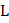# Three identical uniform rods of the same massand lengthare arranged inplane as shown in fig. A fourth uniform rod of mass 3has been placed as shown in theplane. What should be the value of the length of the fourth rod such that the centre of mass of all the four rods lie at the origin?a) 3b) 2c)(+1)/3 d)(2+1)/3

## Question ID - 100215 :- Three identical uniform rods of the same massand lengthare arranged inplane as shown in fig. A fourth uniform rod of mass 3has been placed as shown in theplane. What should be the value of the length of the fourth rod such that the centre of mass of all the four rods lie at the origin?a) 3b) 2c)(+1)/3 d)(2+1)/3

3537

(c)Next Question :
 A particle of mass 2 kg moving with a velocity of 3 m/s is acted upon by a force which changes its direction of motion by an angle ofwithout changing its speed. What is the magnitude of impulse experienced by the particle? a) 6 N s b) 2 N s c) 3N s d) 6N s